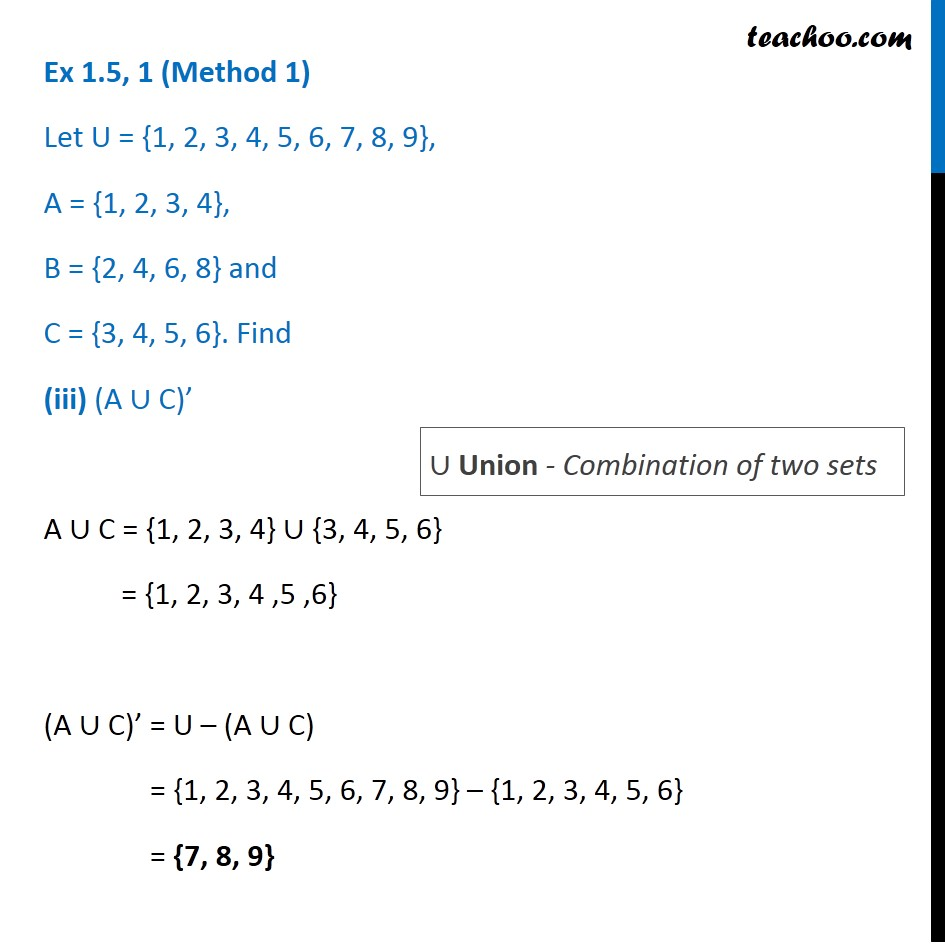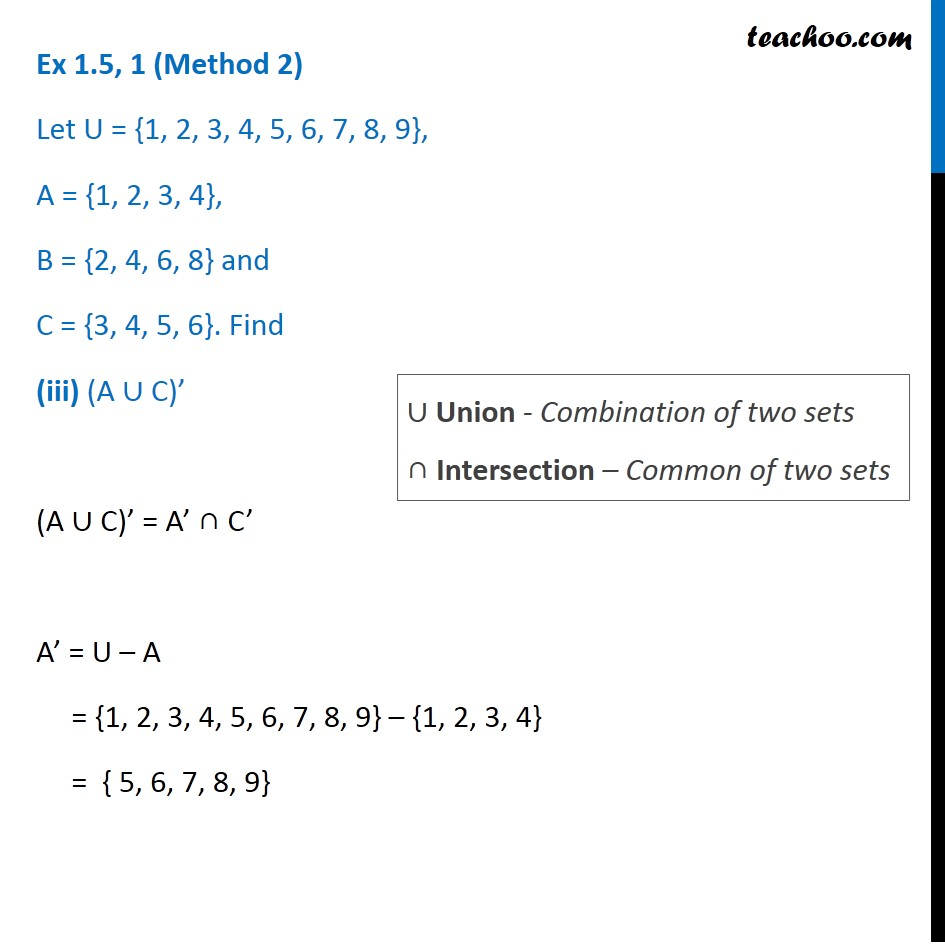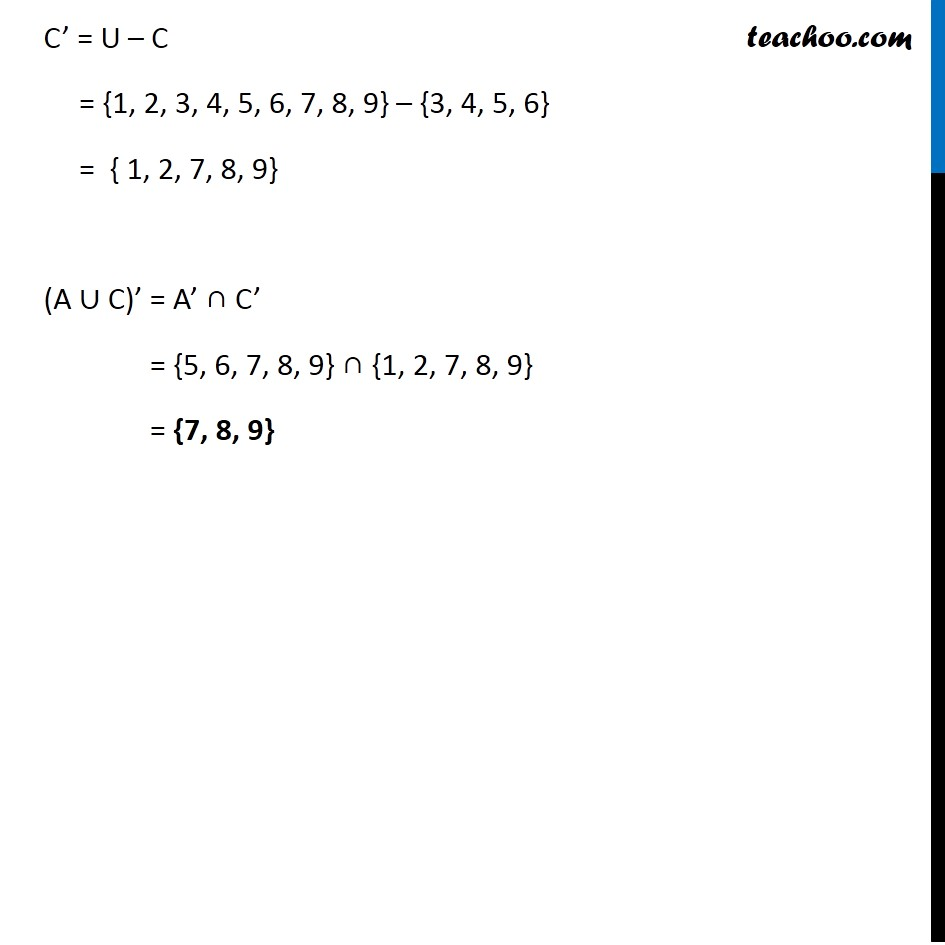1. Chapter 1 Class 11 Sets (Term 1)
2. Serial order wise
3. Ex 1.5

Transcript

Ex 1.5, 1 (Method 1) Let U = {1, 2, 3, 4, 5, 6, 7, 8, 9}, A = {1, 2, 3, 4}, B = {2, 4, 6, 8} and C = {3, 4, 5, 6}. Find (A ∪ C)’ A ∪ C = {1, 2, 3, 4} ∪ {3, 4, 5, 6} = {1, 2, 3, 4 ,5 ,6} (A ∪ C)’ = U – (A ∪ C) = {1, 2, 3, 4, 5, 6, 7, 8, 9} – {1, 2, 3, 4, 5, 6} = {7, 8, 9} ∪ Union - Combination of two sets Ex 1.5, 1 (Method 2) Let U = {1, 2, 3, 4, 5, 6, 7, 8, 9}, A = {1, 2, 3, 4}, B = {2, 4, 6, 8} and C = {3, 4, 5, 6}. Find (A ∪ C)’ (A ∪ C)’ = A’ ∩ C’ A’ = U – A = {1, 2, 3, 4, 5, 6, 7, 8, 9} – {1, 2, 3, 4} = { 5, 6, 7, 8, 9} ∪ Union - Combination of two sets ∩ Intersection – Common of two sets C’ = U – C = {1, 2, 3, 4, 5, 6, 7, 8, 9} – {3, 4, 5, 6} = { 1, 2, 7, 8, 9} (A ∪ C)’ = A’ ∩ C’ = {5, 6, 7, 8, 9} ∩ {1, 2, 7, 8, 9} = {7, 8, 9}